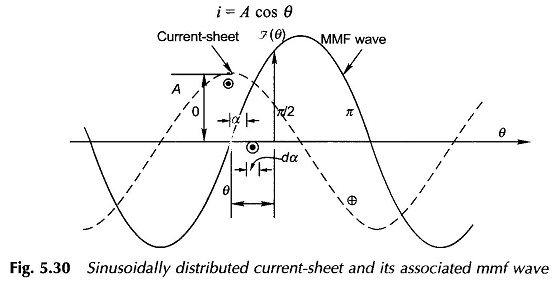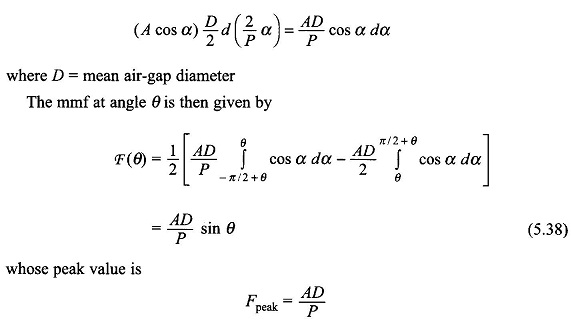## Sinusoidally Distributed Current Sheet Concept:

It was seen above that a distributed winding gives rise to a stepped mmf wave having a strong fundamental component which will be considered in machine modelling while all the harmonic components will be neglected (justification for the same has been advanced).

Now the kind of space distribution of current on the stator (or rotor) has to be found which will produce a purely sinusoidally distributed mmf wave. An intuitive answer is that it would be a Sinusoidally Distributed Current.

Figure 5.30 shows a sinusoidally distributed Current sheet with a peak linear density of A A/m length of the air-gap periphery. The current flows from one end of the stator (or rotor) to the other end parallel to the stator axis. With respect to the angle reference shown, the current distribution is given byThe mmf at angle θ is contributed by the current contained in angular spreads of π/2 on either side of it. The current in the differential element at angle α isAs per Eqs. (5.38) and (5.39), the mmf wave associated with the Sinusoidally Distributed Current-Sheet is also sinusoidal but displaced 90° (elect.) from the current-sheet. Also the peak value of the mmf wave equals half the current contained in one loop of the current-sheet, i.e.

Scroll to Top